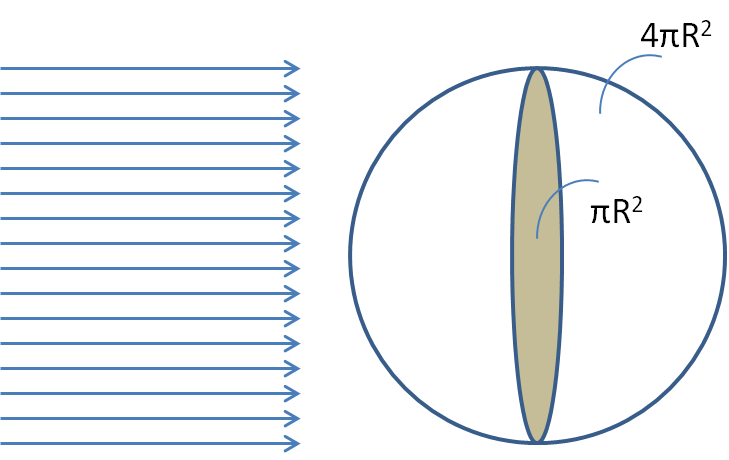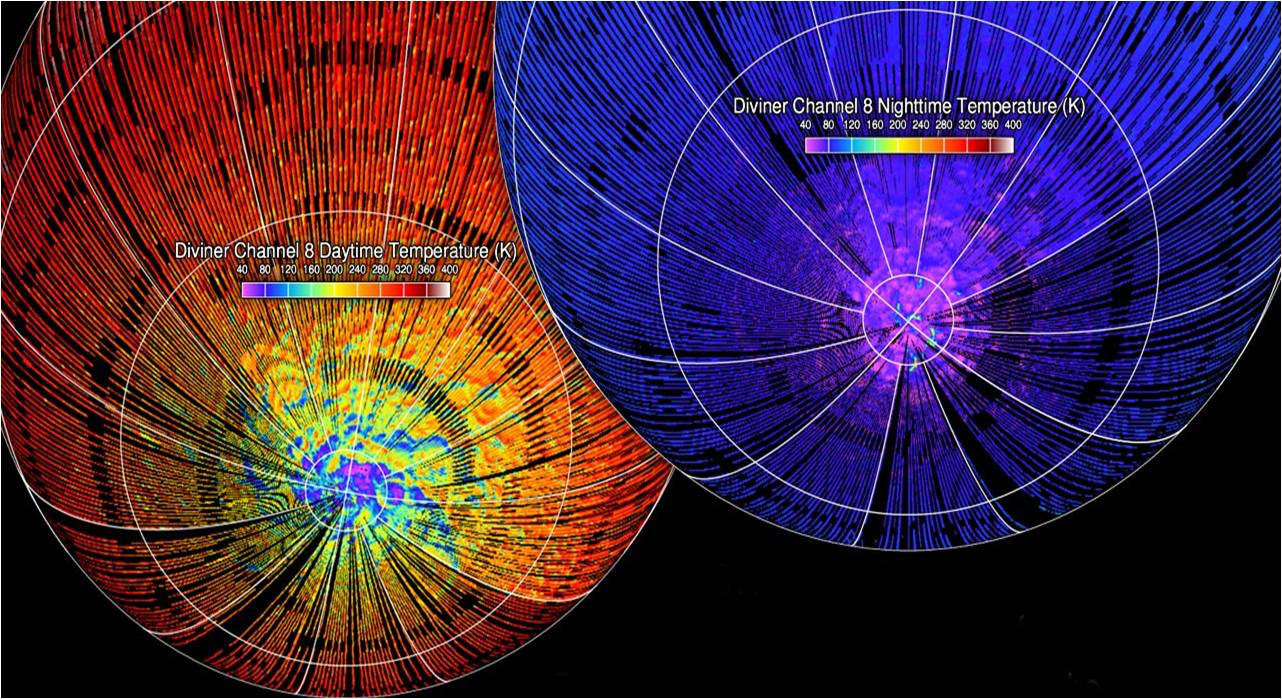## Section23.5Thermal Energy Balance

When a body is in thermal equilibrium with another body, the temperature of the two bodies must be equal, and there would be zero net heat transfer from one body to the other. Two bodies at different temperatures will have a heat transfer from the hotter body to the colder body, causing the temperature of the colder body to rise and that of the hotter body to decrease until their temperatures become equal at the equilibrium.

Would the temperatures of all bodies in thermal contact become equal? Not necessarily, if the body can expend energy to maintain the difference in temperature. For instance, the temperature of our bodies remain quite constant at around $36^{\circ}\textrm{C}$ in environments of different temperatures.

The key is that the loss in heat from our bodies is compensated by the generation of energy within our bodies by biochemical reactions. Therefore, although our bodies are not in thermal equilibrium with the environment, they are in a steady state of nearly constant temperature. The idea of the supply of energy to compensate for the heat loss by a body, or the removal of energy to compensate for a gain in heat by a body, in order to maintain the temperature of the body is called the thermal energy balance.

Although the Moon gains energy from the Sun, the temperature of the Moon remains quite steady. This happens because of thermal energy balance in the absorption and emission of energy by the Moon as we will illustrate in this example by finding the average temperature of the Moon.Consider the Moon to be a spherical black body of radius $1.74 \times 10^6$ m. The intensity of the sunlight on the Moon is approximately $1.4\ kW/m^2\text{,}$ the same as on the earth. Let $R$ be the radius of the moon, and $S$ the power per unit area incident on the moon from the sun. To simplify out calculations, we will assume the Moon to be a perfect black body so that $\epsilon=\alpha=1\text{.}$

To find the balance that would result between the energy gained and energy lost we look at their mechanisms in the case of the Moon. The energy gained will be from the radiation of the Sun incident on the Moon. Since the effective area of the Moon that intersects the almost parallel rays from the Sun is the are of the cross-sectional area of the Moon, we will multiply the flux of the radiation from the Sun by the area $(\pi R^2)$ as shown in Fig. \ref{fig:example-moon-rays-from-sun}. On the other hand, the radiation emitted from the Moon will be emitted from the surface area of the Moon, not the intersected area. Therefore, the energy lost by radiation emitted will be from the surface area $(4\pi R^2)$ of the moon. The two energies must be equal for the balance. Setting the two rates equal gives

\begin{equation*} \pi R^2 S = \sigma (4\pi R^2) T^4. \end{equation*}

Therefore,

\begin{equation*} T = \left( \frac{S}{4\sigma}\right)^{1/4} = \left( \frac{1.4\times 10^3 \textrm{W/m}^2}{4\times 5.7\times 10^{-8} \textrm{W/m}^2\textrm{K}^4}\right)^{1/4} = 280\ \textrm{K}. \end{equation*}

This is approximately $7^{\circ}$C. The temperature of the Moon actually varies over a wide range as found by NASA's Lunar Reconnaissance Orbiter. See Figure 23.5.2.Figure 23.5.2. NASA's Lunar Reconnaissance Orbiter, an unmanned mission to comprehensively map the entire moon produced this thermal map of the north and south polar regions of the Moon in 2008. The temperature of the Moon ranges from approx. 35 K to 400 K. Photo credit NASA.